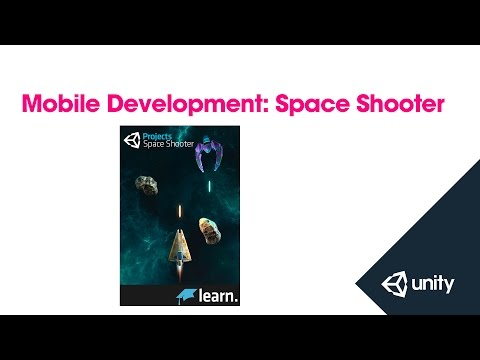# Mobile Development: Converting Space Shooter to Mobile

#### 难度: 新手

In this session we will be taking the Space Shooter tutorial and adding mobile devices to the possible build targets. We will cover touch and accelerometer input, build target defines, and more. Tutor - Adam Buckner## Mobile Development: Converting Space Shooter to Mobile

### Code snippet

``````using UnityEngine;
using UnityEngine.UI;
using UnityEngine.EventSystems;
using System.Collections;

public class SimpleTouchPad : MonoBehaviour, IPointerDownHandler, IDragHandler, IPointerUpHandler {

public float smoothing;

private Vector2 origin;
private Vector2 direction;
private Vector2 smoothDirection;
private bool touched;
private int pointerID;

void Awake () {
direction = Vector2.zero;
touched = false;
}

public void OnPointerDown (PointerEventData data) {
if (!touched) {
touched = true;
pointerID = data.pointerId;
origin = data.position;
}
}

public void OnDrag (PointerEventData data) {
if (data.pointerId == pointerID) {
Vector2 currentPosition = data.position;
Vector2 directionRaw = currentPosition - origin;
direction = directionRaw.normalized;
}
}

public void OnPointerUp (PointerEventData data) {
if (data.pointerId == pointerID) {
direction = Vector3.zero;
touched = false;
}
}

public Vector2 GetDirection () {
smoothDirection = Vector2.MoveTowards (smoothDirection, direction, smoothing);
return smoothDirection;
}
}``````

### Code snippet

``````using UnityEngine;
using UnityEngine.UI;
using UnityEngine.EventSystems;
using System.Collections;

public class SimpleTouchAreaButton : MonoBehaviour, IPointerDownHandler, IPointerUpHandler {

private bool touched;
private int pointerID;
private bool canFire;

void Awake () {
touched = false;
}

public void OnPointerDown (PointerEventData data) {
if (!touched) {
touched = true;
pointerID = data.pointerId;
canFire = true;
}
}

public void OnPointerUp (PointerEventData data) {
if (data.pointerId == pointerID) {
canFire = false;
touched = false;
}
}

public bool CanFire () {
return canFire;
}

}``````

### Code snippet

``````using UnityEngine;
using System.Collections;

[System.Serializable]
public class Done_Boundary
{
public float xMin, xMax, zMin, zMax;
}

public class Done_PlayerController : MonoBehaviour
{
public float speed;
public float tilt;
public Done_Boundary boundary;

public GameObject shot;
public Transform shotSpawn;
public float fireRate;
public SimpleTouchAreaButton areaButton;

private float nextFire;
private Quaternion calibrationQuaternion;

void Start () {
CalibrateAccelerometer ();
}

void Update ()
{
if (areaButton.CanFire () && Time.time > nextFire)
{
nextFire = Time.time + fireRate;
Instantiate(shot, shotSpawn.position, shotSpawn.rotation);
audio.Play ();
}
}

//Used to calibrate the Iput.acceleration input
void CalibrateAccelerometer () {
Vector3 accelerationSnapshot = Input.acceleration;
Quaternion rotateQuaternion = Quaternion.FromToRotation (new Vector3 (0.0f, 0.0f, -1.0f), accelerationSnapshot);
calibrationQuaternion = Quaternion.Inverse (rotateQuaternion);
}

//Get the 'calibrated' value from the Input
Vector3 FixAcceleration (Vector3 acceleration) {
Vector3 fixedAcceleration = calibrationQuaternion * acceleration;
return fixedAcceleration;
}

void FixedUpdate ()
{
//      float moveHorizontal = Input.GetAxis ("Horizontal");
//      float moveVertical = Input.GetAxis ("Vertical");

//      Vector3 movement = new Vector3 (moveHorizontal, 0.0f, moveVertical);

//      Vector3 accelerationRaw = Input.acceleration;
//      Vector3 acceleration = FixAcceleration (accelerationRaw);
//      Vector3 movement = new Vector3 (acceleration.x, 0.0f, acceleration.y);

Vector2 direction = touchPad.GetDirection ();
Vector3 movement = new Vector3 (direction.x, 0.0f, direction.y);
rigidbody.velocity = movement * speed;

rigidbody.position = new Vector3
(
Mathf.Clamp (rigidbody.position.x, boundary.xMin, boundary.xMax),
0.0f,
Mathf.Clamp (rigidbody.position.z, boundary.zMin, boundary.zMax)
);

rigidbody.rotation = Quaternion.Euler (0.0f, 0.0f, rigidbody.velocity.x * -tilt);
}
}``````

### Code snippet

``````using UnityEngine;
using UnityEngine.UI;
using System.Collections;

public class Done_GameController : MonoBehaviour
{
public GameObject[] hazards;
public Vector3 spawnValues;
public int hazardCount;
public float spawnWait;
public float startWait;
public float waveWait;

public Text scoreText;
//  public Text restartText;
public Text gameOverText;
public GameObject restartButton;

private bool gameOver;
//  private bool restart;
private int score;

void Start ()
{
gameOver = false;
//      restart = false;
//      restartText.text = "";
gameOverText.text = "";
restartButton.SetActive (false);
score = 0;
StartCoroutine (SpawnWaves ());
}

//  void Update ()
//  {
//      if (restart)
//      {
//          if (Input.GetKeyDown (KeyCode.R))
//          {
//          }
//      }
//  }

IEnumerator SpawnWaves ()
{
yield return new WaitForSeconds (startWait);
while (true)
{
for (int i = 0; i < hazardCount; i++)
{
GameObject hazard = hazards [Random.Range (0, hazards.Length)];
Vector3 spawnPosition = new Vector3 (Random.Range (-spawnValues.x, spawnValues.x), spawnValues.y, spawnValues.z);
Quaternion spawnRotation = Quaternion.identity;
Instantiate (hazard, spawnPosition, spawnRotation);
yield return new WaitForSeconds (spawnWait);
}
yield return new WaitForSeconds (waveWait);

if (gameOver)
{
restartButton.SetActive (true);
//              restartText.text = "Press 'R' for Restart";
//              restart = true;
break;
}
}
}

public void AddScore (int newScoreValue)
{
score += newScoreValue;
}

{
scoreText.text = "Score: " + score;
}

public void GameOver ()
{
gameOverText.text = "Game Over!";
gameOver = true;
}

public void RestartGame () {